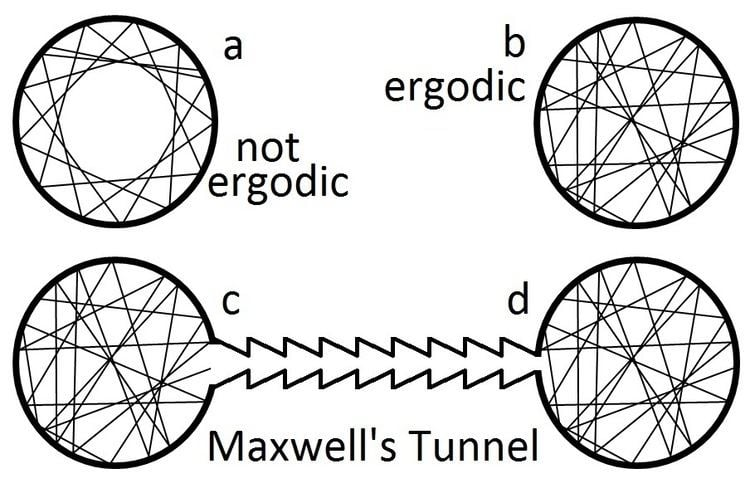# Ergodic hypothesis

Updated on
Covid-19In physics and thermodynamics, the ergodic hypothesis says that, over long periods of time, the time spent by a system in some region of the phase space of microstates with the same energy is proportional to the volume of this region, i.e., that all accessible microstates are equiprobable over a long period of time.

## Contents

Liouville's Theorem states that, for Hamiltonian systems, the local density of microstates following a particle path through phase space is constant as viewed by an observer moving with the ensemble (i.e., the convective time derivative is zero). Thus, if the microstates are uniformly distributed in phase space initially, they will remain so at all times. But Liouville's theorem does not imply that the ergodic hypothesis holds for all Hamiltonian systems.

The ergodic hypothesis is often assumed in the statistical analysis of computational physics. The analyst would assume that the average of a process parameter over time and the average over the statistical ensemble are the same. This assumption that it is as good to simulate a system over a long time as it is to make many independent realizations of the same system is not always correct. (See, for example, the Fermi–Pasta–Ulam experiment of 1953.)

Assumption of the ergodic hypothesis allows proof that certain types of perpetual motion machines of the second kind are impossible. The figure above displays situations where the ergodic hypothesis does and does not hold for a simplistic model of an ideal gas. If the walls are perfectly smooth and circular, the ergodic hypothesis does not hold. If it was possible to construct a sort of tunnel whereby specular reflections cause atoms to move from a less populated container to an identical one with greater density, this would allow the direct conversion of random thermal energy into useful work in a way that does not require a heat bath. But, by Liouville's theorem, if all regions of phase space are equally populated at time, t=0, then they are equally probable for all time. No reflective 'trap' or Maxwell's demon (such as depicted in the figure) will 'unmix' a gas that has randomly filled both containers with equal density and pressure.

## Phenomenology

In macroscopic systems, the timescales over which a system can truly explore the entirety of its own phase space can be sufficiently large that the thermodynamic equilibrium state exhibits some form of ergodicity breaking. A common example is that of spontaneous magnetisation in ferromagnetic systems, whereby below the Curie temperature the system preferentially adopts a non-zero magnetisation even though the ergodic hypothesis would imply that no net magnetisation should exist by virtue of the system exploring all states whose time-averaged magnetisation should be zero. The fact that macroscopic systems often violate the literal form of the ergodic hypothesis is an example of spontaneous symmetry breaking.

However, complex disordered systems such as a spin glass show an even more complicated form of ergodicity breaking where the properties of the thermodynamic equilibrium state seen in practice are much more difficult to predict purely by symmetry arguments. Also conventional glasses (e.g. window glasses) violate ergodicity in a complicated manner. In practice this means that on sufficiently short time scales (e.g. those of parts of seconds, minutes, or a few hours) the systems may behave as solids, i.e. with a positive shear modulus, but on extremely long scales, e.g. over millennia or eons, as liquids, or with two or more time scales and plateaux in between.

## Mathematics

Ergodic theory is a branch of mathematics which deals with dynamical systems that satisfy a version of this hypothesis, phrased in the language of measure theory.

## References

Similar Topics
Daffy Duck and Egghead
Will Peltz
Calum Chambers
Topics

 B i Link H2
 L